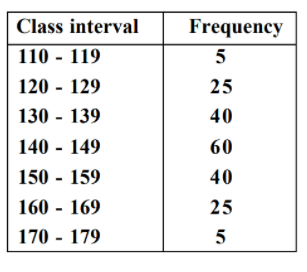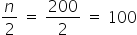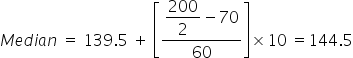Maths-
General
Easy

Question

# The median of the following data is## 134.5    144.5    154.5    140.5Hint:

## The correct answer is: 144.5

### Given :Steps to find median Step 1 : Fix the class interval by subtracting 0.5 from lower limit and adding 0.5 to the upper limit of each interval Class Interval Frequency 109.5 - 119.5 5 119.5 - 129.5 25 129.5 - 139.5 40 139.5 - 149.5 60 149.5 - 159.5 40 159.5 - 169.5 25 169.5 - 179.5 5   n = 200 Step 2: Find the total number of observations(n) = 200Step 3: Define the class size(h), and divide the data into different classes = 10Step 4: Calculate the cumulative frequency of each class. Class Interval Frequency Cumulative Frequency 109.5 - 119.5 5 5 119.5 - 129.5 25 30 129.5 - 139.5 40 70 139.5 - 149.5 60 130 149.5 - 159.5 40 170 159.5 - 169.5 25 195 169.5 - 179.5 5 200   n = 200   Step 5: Identify the class in which the median falls. (Median Class is the class where n/2 lies.)Median class =Thus the median class is 139.5 - 149.5Step 6: Find the lower limit of the median class(l) =  139.5,and the cumulative frequency of the class preceding the median class (c) = 70Now, use the following formula to find the median value.#### With Turito Foundation.#### Get an Expert Advice From Turito.